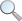e-mail :

Registered in England and Wales company # 03449181.
This website does not use cookies.

Conwy Valley Systems Limited,

West Acre,Llys Helyg,Deganwy

CONWY LL31 9BN, United Kingdom.

VAT# GB 741 5190 48

A Geoscience Wales  founding member.We now have 3 separate definitions of the Trask sorting coefficient. These are:

• As originally given by Trask (1930)
• As reported by Maurice Tucker in his book “Sedimentary Petrology”
• As Tucker but using the square root of (P75/P25) with the percentiles in phi units.

The reason there is more than one definition of the same thing is, first, that it is possible to either  read Trasks’ paper literally or to interpret his intention and, second, that it is easy to overlook the key point of what Trask was doing.

Literally he defines the sorting as the square root of the ratio of the 75th and 25th percentiles, which works because this can be interpreted as an estimate of the spread. Indeed, in a normally distributed data set, the standard deviation is directly related to the percentiles because the central two-thirds of the data set should lie within one standard deviation of the mean; i.e. the range:

[mean minus standard deviation] to [mean plus standard deviation]

should roughly cover the range of values from the 16th percentile to the 84th percentile. Hence simple arithmetic shows that

σ = ½ [P86 – P16]

(where P86  means the grain size which is exceeded only 14 % of the time).

Further, again for a normally distributed data set, the central 95% of the data set should lie within two standard deviations of the mean; i.e. the range:

[mean minus twice the standard deviation] to [mean plus twice the standard deviation]

should roughly cover the range of values from the 2nd  percentile to the 97th percentile (or 3rd to 98th).

Simple arithmetic again shows that

σ = ¼ [P97 – P16]

This isn’t what Trask proposed but does clearly indicate how Folk and Ward derived their estimates of sorting, skewness, etc. The interpretation arises because grain size is rarely normally distributed, but in phi units it is roughly normal. Hence Folk and Ward stipulated that the percentiles should be of the data in phi units. So, maybe Trask was intending us to think of phi-transformed data?

But in his original paper it didn’t matter, because he wasn’t measuring grain sizes, he was counting cumulative weights and inferring grain sizes. This is where, I think, most people using the Trask coefficients have missed the point: using percentiles of the grain sizes gives different results from using percentiles of cumulative weights.

It is easy to make the mistake of thinking (superficially) that it doesn’t matter: if you line up all the grains in order (which is what we’re doing when finding percentiles), then they will be in roughly the same order whether they are ordered by weight or size, and, unless we are distinguishing composition and using accurate density corrections (which of course you can do in PETROG if data were collected using the ‘Concurrent’ option), then they will be in exactly the same order (still not strictly true, because grain size is usually measured by largest diameter, not volume, but if we don’t know the other diameters, then it is effectively the same). The mistake is that Trask didn’t look at individual grains, he just collected grains, by centrifuge, until the weight reached a proportion of the total, and the number of grains remained unspecified and essentially unknowable. So percentiles for Trask were not based on numbers of grains but accumulated weights.

Another reason for interpreting differently is that Trask says:

"if So is less than 2.0, the deposit is well sorted, if it is above 4.5 it is poorly sorted, and if it is about 3.0 it is normally sorted." (‘So’ is his sorting coefficient):

Which does not tally with data calculated using Tucker’s interpretation.

As Tucker says, there is really no need for using Trask’s approximation nowadays, as we can count grains and perform statistical calculations using electronic calculators, but there are two circumstances in which we might want to use it:

1. For comparison. Trask (and this also applies to Folk and Ward) has been used for 85 years, during which time individual geologists have built up a mental picture of what Trask means and laboratories have accumulated a store of data against which new values can be readily compared, and it is in the use as comparison rather than absolute values that the benefit of these analyses lies.
2. Touchstone, the de facto standard clastic reservoir forward modelling system, uses Trask as an input.

For these two reasons, the choice of which of the Trask calculations to use should be dependent less on a notional view of the truth, or on picking through the detail of Trask’s original paper for the correct interpretation, but on how previous Trask values have been calculated. This is why we have chosen to list three different Trask numbers and let the user pick the one that fits in with their requirement, or their company’s past usage, or their client’s requirement, or their model’s requirement.

If you would like to comment on this – for instance, if you think we have interpreted incorrectly – or if you would like us to expand more on our interpretation and provide more mathematical or statistical detail, then please contact us.